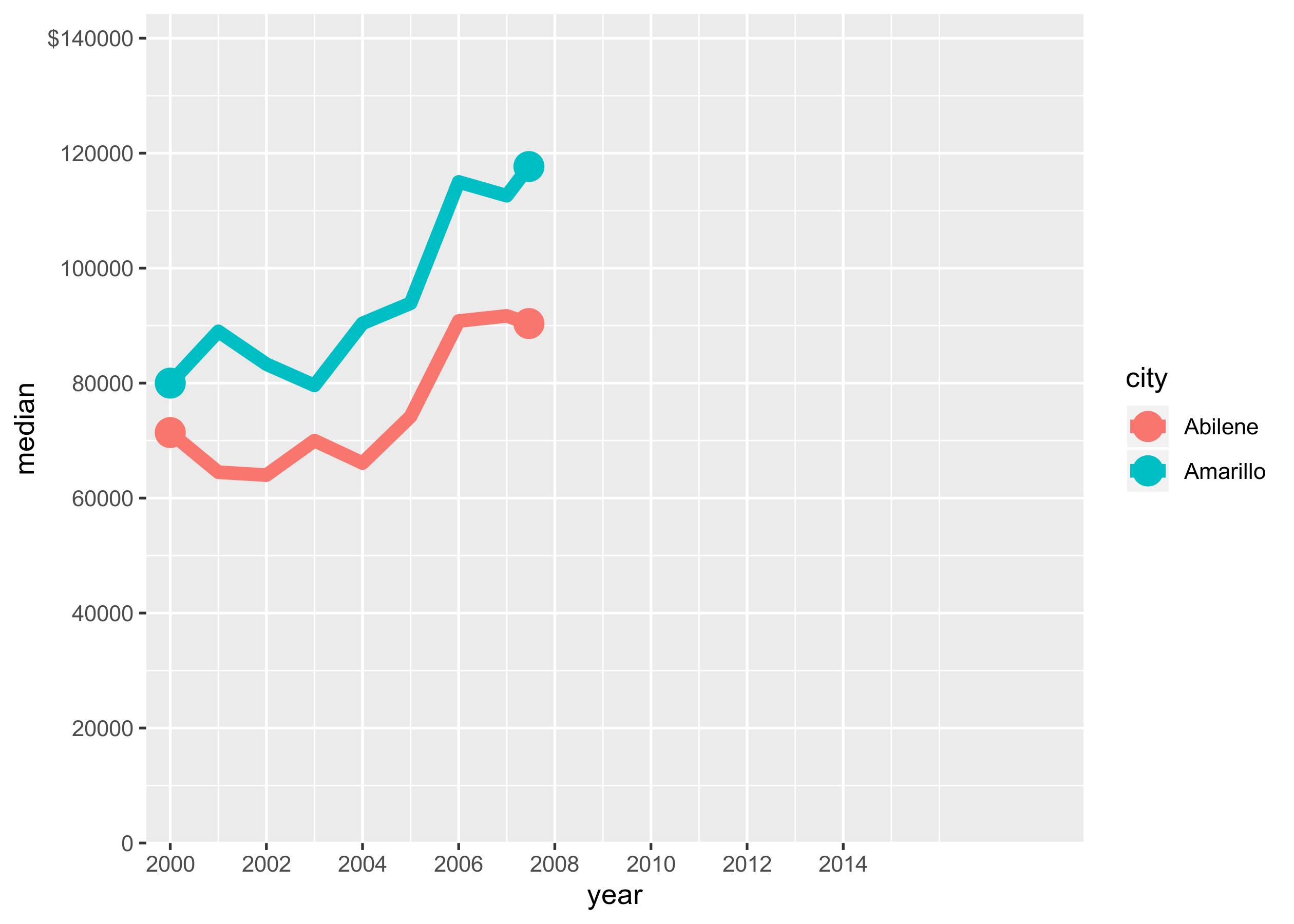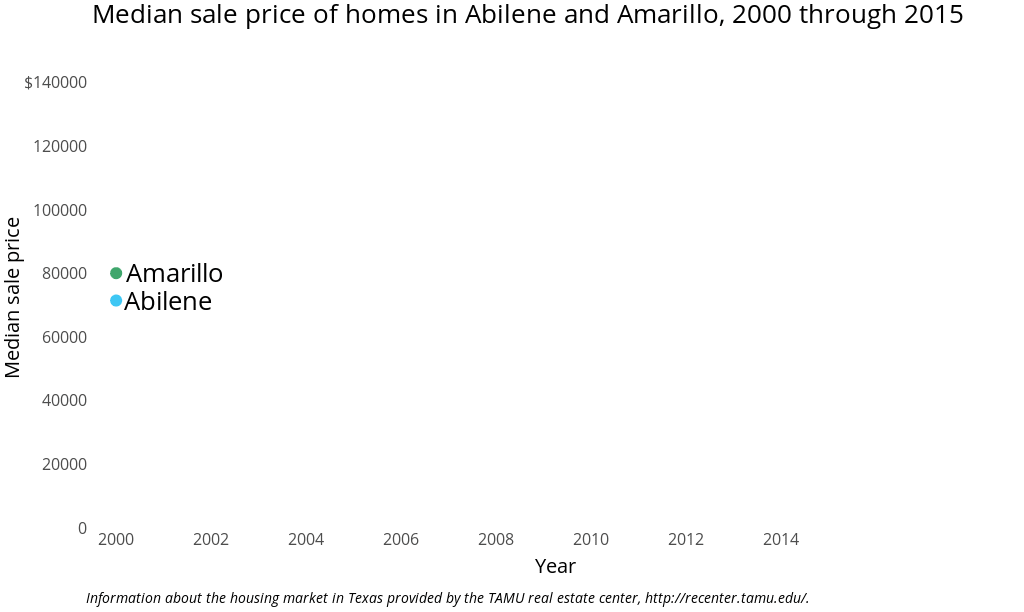# R Animation Tip: Creating Pauses

## Mastering Animation in R

Over the last couple years I’ve developed a multitude of animations using R. During this process I’ve learned quite a bit about R graphics, gpplot2, and useful techniques in animation. In fact I presented on methods for animation in R at satRdays DC - slides available here

One simple technique to improving animations is the addition of pauses. Pauses are an effective method of capturing the viewer’s attention and driving home the main message of an animation. Pausing at the end of an animation (before it loops) offers the viewer the full scope of the visualization, and pauses during the animation can be used to emphasize key data points.

Let’s walk through creating a pause in R.

## Creating Pauses in R - Libraries and Data Reshaping

We’ll use the `txhousing` data set in the `ggplot2` package to demonstrate - this data set contains information regarding the housing market in Texas provided by the TAMU real estate center. The animation will plot the median sale price of two cities - Abilene and Amarillo - from 2000 to 2015.

In my satuRdays DC presentation I covered the steps taken to develop an R animation:

1. Interpolate your data

2. Structure your data to create frames that move across the dimension of interest

3. Determine plot aspects that require consistency across the animation (annotations, scales, legends, etc)

4. Append and export the frames

The variable `year` contains the unique data values across our animation - `split` will generate a list of data frames we can apply interpolations to using the `tweenr` package.

R Libraries and Data Reshaping

``````library(ggplot2)
library(scales)
library(extrafont)
library(dplyr)
library(tweenr)
library(lubridate)
library(animation)
library(RColorBrewer)
library(grid)

# create data frame from txhousing data included in ggplot2 package
txHousingData <- txhousing %>% filter(city %in% unique(txhousing\$city)[1:2] & month==1)
txHousingData <- txHousingData[,c(1,2,6)]
txHousingData\$city <- factor(txHousingData\$city, levels = c("Abilene","Amarillo"), labels = c("Abilene","Amarillo"))

# determine the unique data values that tweenr will iterate over (in this case year)
txList <- split(txHousingData,txHousingData\$year)

# Apply tweenr to interpolate frames between data points
txTweenedData <- tween_states(txList, tweenlength= 1, statelength=0, ease='linear',nframes=200)``````

Since our data is arranged by year by default, it’s already structured in a fashion that moves across our dimension of interest (change over time) - so we’ve already completed the first two animation steps. Now that we’ve got our interpolated data frame `txTweenedData` we can focus on the plot aesthetics.

## Plot Aesthetics

ggplot2 Theme and Color Palette

``````# plot aesthetics
theme_white <- theme(text = element_text(family="Open Sans"),
panel.grid.major.y=element_blank(),
panel.grid.major.x=element_blank(),
panel.grid.minor.x=element_blank(),
panel.grid.minor.y=element_blank(),
axis.title.x=element_text(size=20, margin = margin(t=10)),
axis.title.y=element_text(size=20, margin = margin(t=10)),
axis.text.x=element_text(size=16),
axis.text.y=element_text(size=16),
axis.ticks = element_blank(),
plot.title=element_text(size=26,family = "Open Sans",lineheight=1.15),
plot.subtitle=element_text(size=20, margin = margin(t=20, b = -5),hjust = -0.03, family = "Open Sans"),
plot.caption=element_text(size=14, margin=margin(t=15,r=-15),hjust = -.03,lineheight=1.15, family = "Open Sans", face  = "italic"),
legend.position="none"
)

# color palette
my_palette = c("#3EC7F4", "#3FA66C")``````

It’s critical to determine plot aspects that require consistency across the animation. I like to assign this information as variable objects so that it can easily update across different frames contained in the plotting code. If these aren’t explicitly noted, ggplot2 will render the defaults in their place.

Plot Axes, Labels, and Titles

``````# y axis breaks and labels
yAxisBreaks <- seq(0, 140000, by = 20000)
yAxisLabels <- paste0(format(yAxisBreaks))
yAxisLimits <- c(0,max(yAxisBreaks)* 1.03)
yAxisLabels <- c(yAxisLabels[1:length(yAxisLabels)-1], paste0("\$",yAxisLabels[length(yAxisLabels)]))

# x axis breaks and labels
xAxis <- seq(2000, 2015, by = 2)
xAxisBreaks <- xAxis
xAxisLimits <- c(1999.5, 2019)

# plot text
title <- "Median sale price of homes in Abilene and Amarillo, 2000 through 2015"
subtitle <- ""
caption <- "Information about the housing market in Texas provided by the TAMU real estate center, http://recenter.tamu.edu/."
xLab <- "Year"
yLab <- "Median sale price"

midPoint <- round(max(txTweenedData\$.frame) / 2)

txTweenedData\$LevelLabel <- as.character(txTweenedData\$city)
txTweenedData\$nudge_x <- ifelse(txTweenedData\$LevelLabel=="Abilene", 1.096,ifelse(txTweenedData\$LevelLabel=="Amarillo",1.233,0))
txTweenedData\$nudge_y <- 0``````

## Creating Pauses

Finally we’ve reached our R animation tip - and thankfully it’s pretty trivial! To generate pauses within an animation simply determine where the pause exists, subset your data to include that frame, and use `replicate` and `grid.draw` to repeat that frame n-times. For instance, say I wanted to pause at the midpoint of the animation to emphasize a point. I’d first calculate the midpoint, then ensure that my data includes all frames until that point: `subset(txTweenedData, .frame <= midPoint)`.

Animation Code

``````#calculate midpoint of the plot
midPoint <- round(max(txTweenedData\$.frame) / 2)

g2 <- ggplot(data = subset(txTweenedData, .frame <= midPoint), aes(x = year, y = median, .frame = midPoint)) +
geom_point(data = subset(txTweenedData, .frame == min(.frame)),aes(group=city, color=city), size=5) +
geom_point(data = subset(txTweenedData, .frame == midPoint),aes(group=city, color=city), size=5) +
geom_line(aes(group=city, color=city, cumulative = TRUE, label=LevelLabel), size=2.5) +
scale_x_continuous(labels=xAxisBreaks, expand = c(0, 0), breaks=xAxisBreaks, limits =xAxisLimits) +
scale_y_continuous(labels=yAxisLabels, expand = c(0, 0), breaks=yAxisBreaks,limits = yAxisLimits)
g2``````Once we’ve created this frame, `replicate` and `grid.draw` repeat that frame n-times to create a pause.

``replicate(75,grid.draw(g2))``

Simple as that!

## Full Animation Code within `saveGIF`

(NOTE: click on the gray bars to toggle collapsing the code!)

Animation Code

``````saveGIF({

# print from tue first frame to the midpoint of the figure
for(i in 1:midPoint) {
g1 <-  ggplot(data = subset(txTweenedData, .frame <= i), aes(x = year, y = median, .frame = i)) +
geom_point(data = subset(txTweenedData, .frame == min(.frame)),aes(group=city, color=city), size=5) +
geom_line(aes(group=city, color=city, cumulative = TRUE, label=LevelLabel), size=2.5) +
scale_x_continuous(labels=xAxisBreaks, expand = c(0, 0), breaks=xAxisBreaks, limits =xAxisLimits) +
scale_y_continuous(labels=yAxisLabels, expand = c(0, 0), breaks=yAxisBreaks,limits = yAxisLimits) +
theme_minimal() + theme_white + scale_color_manual(values=my_palette) +
geom_text(data = subset(txTweenedData, .frame == i),aes(label =LevelLabel), size = 9, nudge_y = subset(txTweenedData, .frame == i)\$nudge_y, nudge_x = subset(txTweenedData, .frame == i)\$nudge_x, family = "Open Sans", lineheight = 0.75) +
labs(x=xLab, y=yLab, title = title, subtitle = subtitle,
caption = caption)
print(g1);
}

# print the midpoint 75 times to create a pause
g2 <- ggplot(data = subset(txTweenedData, .frame <= midPoint), aes(x = year, y = median, .frame = midPoint)) +
geom_point(data = subset(txTweenedData, .frame == min(.frame)),aes(group=city, color=city), size=5) +
geom_point(data = subset(txTweenedData, .frame == midPoint),aes(group=city, color=city), size=5) +
geom_line(aes(group=city, color=city, cumulative = TRUE, label=LevelLabel), size=2.5) +
scale_x_continuous(labels=xAxisBreaks, expand = c(0, 0), breaks=xAxisBreaks, limits =xAxisLimits) +
scale_y_continuous(labels=yAxisLabels, expand = c(0, 0), breaks=yAxisBreaks,limits = yAxisLimits) +
theme_minimal() + theme_white + scale_color_manual(values=my_palette) +
geom_text(data = subset(txTweenedData, .frame == midPoint),aes(label =LevelLabel), size = 9, nudge_y = subset(txTweenedData, .frame == midPoint)\$nudge_y, nudge_x = subset(txTweenedData, .frame == midPoint)\$nudge_x, family = "Open Sans", lineheight = 0.75) +
labs(x=xLab, y=yLab, title = title, subtitle = subtitle,
caption = caption)

replicate(75,grid.draw(g2))

# print from tue midpoint of the figure until the last frame
for(i in midPoint:max(txTweenedData\$.frame)) {
g3 <- ggplot(data = subset(txTweenedData, .frame <= i), aes(x = year, y = median, .frame = i)) +
geom_point(data = subset(txTweenedData, .frame == min(.frame)),aes(group=city, color=city), size=5) +
geom_line(aes(group=city, color=city, cumulative = TRUE, label=LevelLabel), size=2.5) +
scale_x_continuous(labels=xAxisBreaks, expand = c(0, 0), breaks=xAxisBreaks, limits =xAxisLimits) +
scale_y_continuous(labels=yAxisLabels, expand = c(0, 0), breaks=yAxisBreaks,limits = yAxisLimits) +
theme_minimal() + theme_white + scale_color_manual(values=my_palette) +
geom_text(data = subset(txTweenedData, .frame == i),aes(label =LevelLabel), size = 9, nudge_y = subset(txTweenedData, .frame == i)\$nudge_y, nudge_x = subset(txTweenedData, .frame == i)\$nudge_x, family = "Open Sans", lineheight = 0.75) +
labs(x=xLab, y=yLab, title = title, subtitle = subtitle,
caption = caption)
print(g3);  }

# print the final frame of the figure 110 times to create an ending pause
wholeFig <- ggplot(data = subset(txTweenedData, .frame <= i), aes(x = year, y = median, .frame = i)) +
geom_point(data = subset(txTweenedData, .frame == min(.frame)),aes(group=city, color=city), size=5) +
geom_point(data = subset(txTweenedData, .frame == i),aes(group=city, color=city), size=5) +
geom_line(aes(group=city, color=city, cumulative = TRUE, label=LevelLabel), size=2.5) +
scale_x_continuous(labels=xAxisBreaks, expand = c(0, 0), breaks=xAxisBreaks, limits =xAxisLimits) +
scale_y_continuous(labels=yAxisLabels, expand = c(0, 0), breaks=yAxisBreaks,limits = yAxisLimits) +
theme_minimal() + theme_white + scale_color_manual(values=my_palette) +
geom_text(data = subset(txTweenedData, .frame == i),aes(label =LevelLabel), size = 9, nudge_y = subset(txTweenedData, .frame == i)\$nudge_y, nudge_x = subset(txTweenedData, .frame == i)\$nudge_x, family = "Open Sans", lineheight = 0.75) +
labs(x=xLab, y=yLab, title = title, subtitle = subtitle,
caption = caption)

replicate(110,grid.draw(wholeFig))
},movie.name="/home/michael/Documents/animation_samples/txHousing/txHousing.gif",interval = .02, ani.width = 1024, ani.height = 612)``````Median sale price of homes in Abilene and Amarillo, 2000 through 2015

Finally it’s smart to compress the final animation, since gifs can be unwieldy.

GIF Compression

``````# compress gif
gif_compress <- function(ingif, outgif, show=TRUE, extra.opts=""){
command <-  sprintf("gifsicle -O3 %s < %s > %s", extra.opts, ingif, outgif)
system.fun <- if (.Platform\$OS.type == "windows") shell else system
if(show) message("Executing: ", strwrap(command, exdent = 2, prefix = "\n"))
system.fun(ifelse(.Platform\$OS.type == "windows", sprintf("\"%s\"", shQuote(command)), command))
}

gif_compress("/home/michael/Documents/animation_samples/txHousing/txHousing.gif","/home/michael/Documents/animation_samples/txHousing/tx_Housing.gif",extra.opts="--colors 256")``````

Hope you’ve found this useful! Feel free to reach out to me on twitter with any questions or feedback - https://twitter.com/mikeleeco